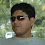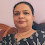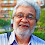## Wednesday, January 11, 2012

### Around the World

Theres a playground near my house. It is circular and about the size of a football or cricket field. I usually cycle on the track that runs around the edge of the ground. I once did a rough measurement and its radius was around 63 metres. Doing such a rough measurement is simple. I just started from the center and counted how many times I had to pedal do reach the edge. I do around 1.5 meters on a full turn of my pedal... so it is easy to get a good enough measure of the radius.

The Earth is around 100000 times larger than this playground. Earth's radius is around 6300 km (1 km = 1000 metres). That means if someone cycled on a track around the equator, they would be covering 100000 times what I do in the playground! It is impossible for any one to do it though. May be we could do it if we were birds.

There is a road that runs outside this playground. I sometimes cycle on that as well. The road runs all round outside the playground, around 7 meters away from it. I figured that because the radius of this road is 7 metres more than that of the playground, I was cycling 44 metres more for every full circle on the road than in the playground. We all know it... larger circle and hence larger circumference.

Now here's the question... We know that:

• If I cycle 7m away from the playground track, I cycle 44m more than what I would by cycling on the track.
• The equator in 100000 times larger than my playground.

So if a bird flies 7m above ground around the equator, how much more distance it will cover than what it would if it hopped along on the ground?

I'm sure you can answer it and you may find it amusing. I'll put up the answer in a few days. In the meantime, if you know the answer, do put it up as a comment!

Ok... So let us try to understand the matter.
The circumference of a circle varies with its radius (roughly 6 times) by the formula:
circumference = 2 * pi * radius, where pi is the magic number 22 / 7

With this information, lets see how myself and the bird fare when we both increase our path radius by 7m.

Me on the playground:
Radius of playground = 63 m
Circumference = 2 * (22 / 7) * 63 = 2 * 22 * 9 = 396 m
Circumference of road = 2 * (22 / 7) * (63 + 7) = 396 + (2 * 22) = 396 + 44 m
Difference in circumference = 44 m

Bird flying around the earth:
Radius of earth = 6300000 m
Circumference = 2 * (22 / 7) * 6300000 = 2 * 22 * 900000 = 39600000 m
So a bird hopping along on the ground will cover 39600000 m.
Radius that is 7m above the ground = (6300000 + 7) m
Circumference of circle 7m above ground = 2 * (22 / 7) * (6300000 + 7) = 39600000 + 44 m
So a bird flying 7m above ground will cover (39600000 + 44) m.
Difference in circumference = 44 m

So, it does not matter whether it is me cycling around a track or a bird flying around the world. The length of our paths may be very different, but if we increase the radius of our paths by 7m we cover the same 44m additional distance.

1.what an observation ?
you see everything with a science eye !
great thinking ;0
DeePaK

2.nice mind... what's the ans ? -Rishi

3.Can't say that it matters to me :))

4.still you din't put up ???
awaiting and checking :P

1.Thanks for checking back! The answer is out now!

5.it will cover the same dist i guess coz its encircling the same dist around the equator whether its flying or hopping....might b wrng :P :D..
yet i tried :)

do visit my blog:
http://campbuzzz.blogspot.com/2012/01/youngistan.html

1.Thanks for trying.

6.Too tired to think of answer today... let me know when you put it up ;)

1.7.the Circumference of the ground is 395 m
the circumference of the road is 439 m
therefore the Circumference of the earth is 39500000 m
and the bird flies, 43900000 m if it flies 7 meters above the ground
the diff is 4400000 m I think :-)

1.That was great Santa. I think you almost got it. It's just that 7m above ground makes the radius (395*100000 + 7) and not (395 + 7)*100000. That's a mistake even I did when I didn't think too carefully. Thanks.

8.Thank you. I'm all ears!